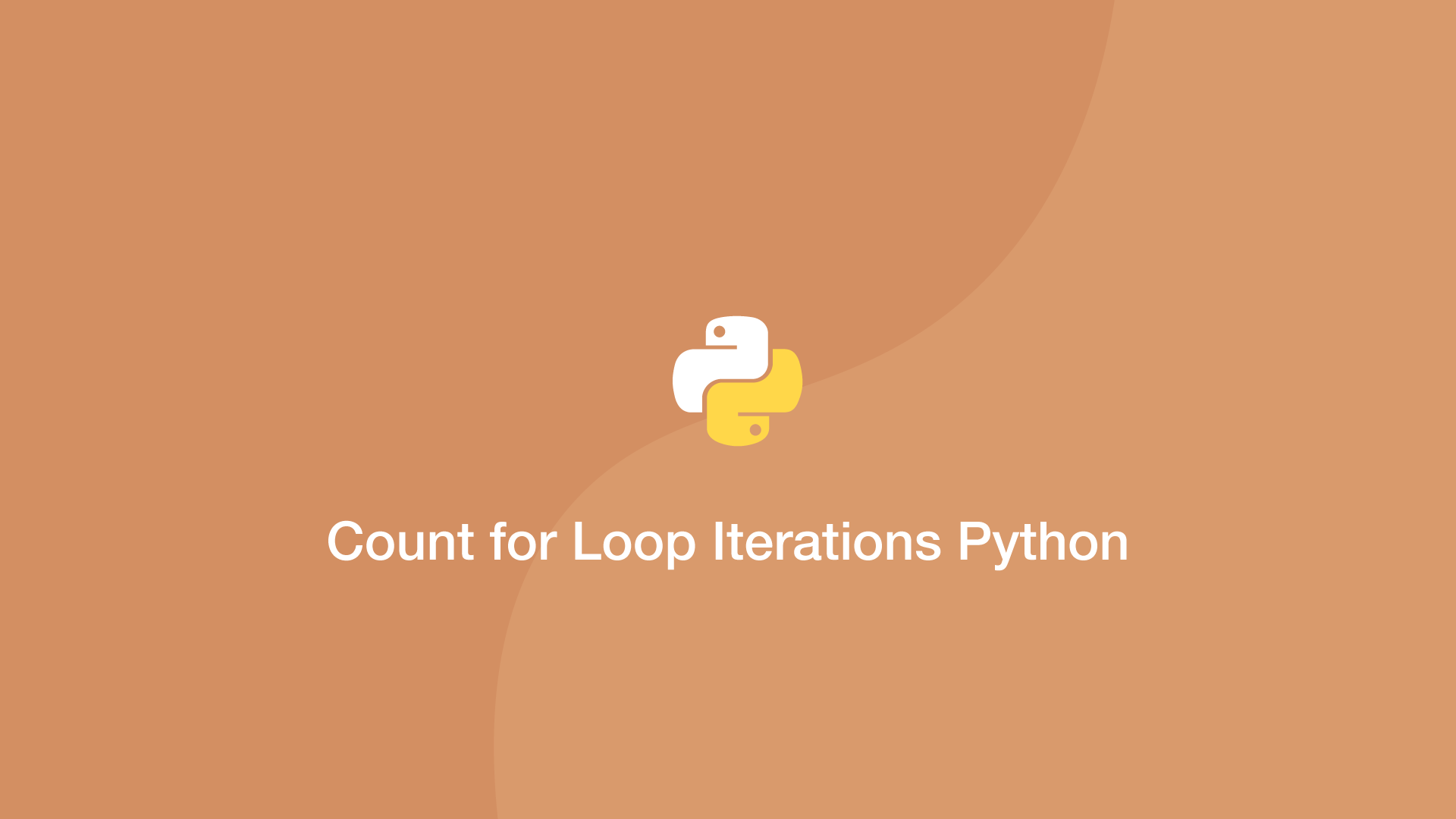# How to Count the Number of for Loop Iterations in PythonIn this tutorial, we will learn how to count each iteration in a Python `for` loop for a final count or to use the current iteration number inside the loop.

To count iterations we can use the Python `enumerate()` function and pass it in as the first argument of the `for` loop.

``````items = ["a", "b", "c", "d"]

for iteration, item in enumerate(items):
print(iteration)
``````
``````0
1
2
3
``````

## Get Total Number of Iterations

If you only need the total iterations that were made, you can increment an integer by 1 on each iteration.

``````items = ["a", "b", "c", "d"]
cnt = 0

for item in items:
cnt += 1

print(cnt)
``````
``````4
``````

#### Related Tutorials### How to Use For Loops in Python

September 02, 2020### How to Use While Loops in Python

September 03, 2020### How to Create and Use Arrays in JavaScript

July 11, 2020### How to Calculate Mean, Median, Mode and Range in Python

October 07, 2020### How to Enumerate in Python

September 17, 2020### How to Create and Use Lists (Arrays) in Python

September 01, 2020